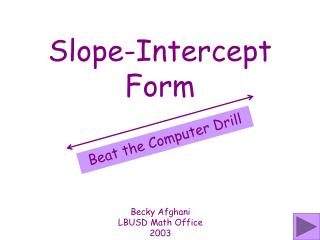DownloadDownload PresentationSlope-Intercept Form

# Slope-Intercept Form

Download Presentation## Slope-Intercept Form

- - - - - - - - - - - - - - - - - - - - - - - - - - - E N D - - - - - - - - - - - - - - - - - - - - - - - - - - -
##### Presentation Transcript

1. Slope-Intercept Form Beat the Computer Drill Becky Afghani LBUSD Math Office 2003

2. Directions A slide will appear showing the graph of a line. Say the equation for the line in y = mx + b form aloud before the computer can answer (6 sec). BEAT THE COMPUTER! You will hear a sound when the slide changes to a new problem.

3. Y = 5x - 2

4. y = -2x

5. 4 3 y = x - 1

6. y = 1

7. 2 3 y = x

8. y = x

9. 1 4 y = x

10. y = -6x - 3

11. x = 2

12. y = 6x - 3

13. y = 3x

14. 1 2 y = x

15. 5 2 y = x - 3

16. 1 3 y = x

17. y = -4x + 2

18. 2 3 y = x

19. 4 3 y = x - 1

20. y = 4x - 2

21. 5 3 y = x - 2

22. 1 4 y = x

23. y = -5x - 2

24. 5 3 y = x - 2

25. 3 2 y = x

26. y = -x